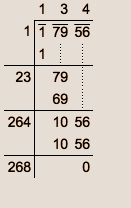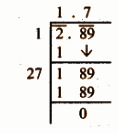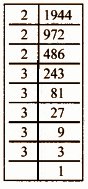# Samacheer Kalvi 8th Maths Book Solutions Term 3 Chapter 1 | Numbers Answers For Tamil Nadu Board

Samacheer Kalvi 8th Maths Book Solutions Term 3 Chapter 1 – Numbers is available here. The Samacheer Kalvi 8th Maths book answers of Term 3 Chapter 1, available at BYJU’S, contain step by step explanations designed by our Mathematics experts. All these important questions are based on the new pattern prescribed by the Tamil Nadu board. Students can also get the solutions of other chapters on Samacheer Kalvi 8th Maths solutions. Referring to these Samacheer Kalvi Class 8 Maths textbook  Term 3 Chapter 1 questions and solutions is the best way for the students to self-gauge their performance and exam preparation level.

Term 3 Chapter 1 of the Samacheer Kalvi 8th Maths guide will help the students to solve problems related to square numbers, Pythagorean triplets, square root and finding them through different methods, square root of decimal numbers, cubes and cube numbers, properties of cubes of numbers, cube root, exponents and powers, laws of exponents, standard notation and scientific notation.

### Samacheer Kalvi 8th Maths Term 3 Chapter 1: Numbers Book Exercise 1.1 Questions and Solutions

Question 1: Write

(i) 102 and

(ii) 112 as the sum of consecutive odd natural numbers.

Solution:

(i) 102

= 100

= 1 + 3 + 5 + 7 + 9 + 11 + 13 + 15 + 17 + 19

(ii) 112

= 121

= 1 + 3 + 5 + 7 + 9 + 11 + 13 + 15 + 17 + 19 + 21

Question 2: Find a Pythagorean triplet whose

(i) the largest member is 65

(ii) the smallest member is 10

Solution:

(i) the largest member is 65

2m, m2 – 1, m2 + 1 form a Pythagorean triplet

Let m2 + 1 = 65

m2 = 65 – 1

m2 = 64

m2 = 82

m = 8

2m = 2 * 8 = 16

m2 – 1 = 82 – 1 = 63

The Pyathgorean triplet is (16, 63, 65).

(ii) the smallest member is 10

2m, m2 – 1, m2 + 1 form a Pythagorean triplet

Let 2m = 10

m = 10 / 2

m = 5

m2 – 1 = 52 – 1 = 24

m2 + 1 = 52 + 1 = 26

The Pythagorean triplet is (10, 24, 26).

### Samacheer Kalvi 8th Maths Term 3 Chapter 1: Numbers Book Exercise 1.2 Questions and Solutions

Question 1: Find the square root by long division method: 17956

Solution:Number = 17956

Square Root = 134

Question 2: Find the square root of the decimal number: 2.89.

Solution:Decimal = 2.89

Square root = 1.7

### Samacheer Kalvi 8th Maths Term 3 Chapter 1: Numbers Book Exercise 1.3 Questions and Solutions

Question 1: Show that 1944 is not a perfect cube.

Solution:1944 = 2 * 2 * 2 * 3 * 3 * 3 * 3 * 3

= 23 * 35

= 23 * 33 * 3 * 3

There are two triplets. To make further triplets, 1944 has to be multiplied with 3.

So, 1944 is not a perfect cube.

Question 2: Find two smallest perfect square numbers which when multiplied together gives a perfect cube number.

Solution:

Consider two numbers 4 and 16.

The product of the numbers is 4 * 16 = 64

64 = 4 * 4 * 4

The required square numbers are 4 and 16.

### Samacheer Kalvi 8th Maths Term 3 Chapter 1: Numbers Book Exercise 1.4 Questions and Solutions

Question 1: Find the number in standard form: 8 * 104 + 7 * 103 + 6 * 102 + 5 * 101 + 2 * 1 + 4 * 10-2 + 7 * 10-4.

Solution:

8 * 104 + 7 * 103 + 6 * 102 + 5 * 101 + 2 * 1 + 4 * 10-2 + 7 * 10-4

= 8 * 10000 + 7 * 1000 + 6 * 100 + 5 * 10 + 2 * 1 + 4 * (1 / 100) + 7 * (1 / 10000)

= 80000 + 7000 + 600 + 50 + 2 + (4 / 100) + (7 / 10000)

= 87652.0407

Question 2: The radius of a hydrogen atom is 2.5 × 10–11 m. Express this number in standard notation.

Solution:

The radius of a hydrogen atom is 2.5 × 10–11 m

= 2.5 * (1 / 1011) m

= (2.5 / 1011) m

= 0.000000000025 m

### Samacheer Kalvi 8th Maths Term 3 Chapter 1: Numbers Book Exercise 1.5 Questions and Solutions

Question 1: If 2m-1 + 2m+1 = 640, then find m.

Solution:

2m-1 + 2m+1 = 640

2m-1 + 2m+1 = 128 + 512

2m-1 + 2m+1 = 27 + 29

m – 1 = 7

m + 1 = 9

m = 7 + 1

m = 8

Question 2: Give the answer in scientific notation: A human heart beats at an average of 80 beats per minute. How many times does it beat in

(i) an hour?

(ii) a day?

Solution:

(i) an hour

Heartbeat per minute = 80 beats

1 hour = 60 minutes

Heartbeat in an hour = 80 * 60

= 4800

= 4.8 * 103

(ii) a day

1 day = 24 hours = 24 * 60 minutes

Heartbeat per minute = 80 beats

Heartbeat in a day = 24 * 60 * 80

= 24 * 4800

= 115200

= 1.152 * 105